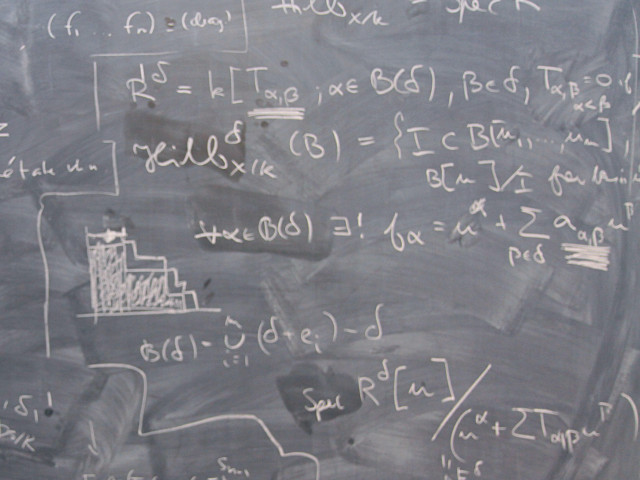# SF1906 Mathematical Statistics, Basic Course 9.0 credits

#### Matematisk statistik, grundkursThe overall purpose of the course is that the student should be well acquainted with basic concepts, theory, models and solution methods in probability theory and statistical inference.

### Offering and execution

Course offering missing for current semester as well as for previous and coming semesters

## Course information

### Content and learning outcomes

#### Course contents *

Basic concepts like probabilities, conditional probabilities and independent events. Discrete and continuous random variables, especially one dimensional random variables. Measures of location, scale and dependency of random variables and data sets. Common distributions and models: normal, binomial and Poisson distribution. Central limit theorem and Law of large numbers.

Descriptive statistics.

Point estimates and general methods of estimation as the method of maximum likelihood and least squares. General confidence intervals but specifically confidence intervals for mean and variance of normally distributed observations. Confidence intervals for proportions, difference in means and proportions.

Testing statistical hypothesis. Chi2-test of distribution, test of homogeneity and contingency. Linear regression.

Markov processes with discrete state spaces. Absorption, stationarity and ergodicity of Markov chains. Properties of birth and death processes in general and Poisson process in particular. Standard queueing models M/M/1 and M/M/c and queueing theory.

#### Intended learning outcomes *

To pass the course, the student should be able to do the following:

• construct elementary statistical models for experiments
• describe standard models and explain the applicability of the models in given examples
• define and calculate descriptive quantities like expectation, variance, and percentiles for distributions and data sets.
• with standard methods calculate estimates of unknown quantities and quantify the uncertainty in these estimates
• value and compare methods of estimation
• analyse how measuring accuracy affect conclusions and quantify risks and error probabilities in statistical analysis
• construct simple Markov chain models in discrete and continuous time and describe their asymptotic properties and behaviour, in particular the Poisson process
• use absorption techniques in continuous and discrete time Markov chains to solve problems
• model simple queueing systems with birth and death processes and calculate theoretical quantities in these models such as expected queueing time and queue length.

• Combine all the concepts and methods mentioned above in order to solve more complex problems.

#### Course Disposition

No information inserted

### Literature and preparations

#### Specific prerequisites *

Basic courses in differential and integral calculus. Basic course in linear algebra.

#### Recommended prerequisites

No information inserted

#### Equipment

No information inserted

#### Literature

Blom et al. Sannolikhetslära och statistikteori med tillämpningar. Studentlitteratur.
Complemental material from the department.

### Examination and completion

A, B, C, D, E, FX, F

#### Examination *

• TEN1 - Examination, 6.0 credits, Grading scale: A, B, C, D, E, FX, F
• ÖVN1 - Assignments, 3.0 credits, Grading scale: P, F

Based on recommendation from KTH’s coordinator for disabilities, the examiner will decide how to adapt an examination for students with documented disability.

The examiner may apply another examination format when re-examining individual students.

#### Opportunity to complete the requirements via supplementary examination

No information inserted

#### Opportunity to raise an approved grade via renewed examination

No information inserted

#### Examiner

No information inserted

### Further information

#### Course web

No information inserted

SCI/Mathematics

#### Main field of study *

Mathematics, Technology

First cycle